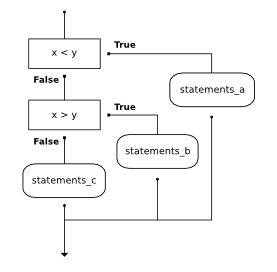# 3. Conditionals¶

Quick Overview of Day

Go over practice problems from last day. Give more details about boolean expressions. Practice some Python problems with input/output and conditionals.

## 3.1. What Does This Program Do?¶

Remember that in our quick Python overview, we saw that a single equal sign `=` is used to assign a value. Two equal signs `==` are used when comparing a value.

Note

Your teacher may choose to use the following examples as a class activity, by displaying the examples, and having you take a guess as to what you think each will do before running the code.

What will the following programs output? Why?

## 3.2. Booleans¶

The Python type for storing true and false values is called `bool`, named after the British mathematician, George Boole. George Boole created Boolean Algebra, which is the basis of all modern computer arithmetic.

There are only two boolean values. They are `True` and `False`. Capitalization is important, since `true` and `false` are not boolean values (remember Python is case sensitive).

Note

Boolean values are not strings!

It is extremely important to realize that True and False are not strings. They are not surrounded by quotes. They are the only two values in the data type `bool`. Take a close look at the types shown below.

A boolean expression is an expression that evaluates to a boolean value. The equality operator, `==`, compares two values and produces a boolean value related to whether the two values are equal to one another.

In the first statement, the two operands are equal, so the expression evaluates to `True`. In the second statement, 5 is not equal to 6, so we get `False`.

The `==` operator is one of six common comparison operators; the others are:

```x != y               # x is not equal to y
x > y                # x is greater than y
x < y                # x is less than y
x >= y               # x is greater than or equal to y
x <= y               # x is less than or equal to y
```

We have already been using most of these, but `!=` is new to us. You should also remember that we used `not` with Reeborg, and that `not` switches the value of a boolean expression. Consider the following:

When asking the computer a question with a boolean expression, a common error is to use a single equal sign (`=`) instead of a double equal sign (`==`). Remember that `=` is an assignment operator and `==` is a comparison operator.

## 3.3. Practice Problems¶

Try the following practice problems. You can either work directly in the textbook, or using Thonny. Either way, be sure to save your solution into your Computer Science 20 folder when you finish!

Note

Remember that every time you take `input()` from the user, the data type of that input will be a string! Sometimes you need to convert what the user enters into a number.

Write a program that can either add or subtract two numbers. You should first ask the user whether they want to add or subtract, then take in the two numbers, then finally perform the required operation and print the result.

Do not look at this sample solution unless you have already finished creating your own solution!

### 3.3.2. Area Calculator¶

Write a program that asks the user if they want to find the area of a rectangle, circle, or triangle. Then have the user input the appropriate sizes (length and width, radius, or base and height) for the shape you will be calculating. Finally, perform the calculation and output the result with a nice message. Note: You might want to use the code you created yesterday to help you create parts of this!

## 3.4. If You Are Having Trouble - More Details on Conditionals¶

### 3.4.1. `if/else`¶

The `if`, `if/else` and `if/elif/else` control structures are all referred to as conditional statements. Note that each time you ask the computer a question using one of these conditional statements, Python evaluates the question as a Boolean expression.

Just like with Reeborg, the syntax for an `if` statement looks like this:

```if BOOLEAN EXPRESSION:
STATEMENTS_1        # executed if condition evaluates to True
else:
STATEMENTS_2        # executed if condition evaluates to False
```

The boolean expression after the `if` statement is called the condition. If it is true, then the immediately following indented statements get executed. If not, then the statements indented under the `else` clause get executed.

The more indented statements that follow are called a block. There is no limit on the number of statements that can appear under the two clauses of an `if` statement, but there has to be at least one statement in each block.

### 3.4.2. `if`¶

Another form of the `if` statement is one in which the `else` clause is omitted entirely. This creates what is sometimes called unary selection. In this case, when the condition evaluates to `True`, the statements are executed. Otherwise the flow of execution continues to the statement after the body of the `if`.

What would be printed if the value of `x` is negative? Try it.

### 3.4.3. `if/elif/else`¶
`elif` is an abbreviation of `else if`. Remember that exactly one branch will be executed. There is no limit of the number of `elif` statements but only a single (and optional) final `else` statement is allowed and it must be the last branch in the statement.